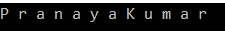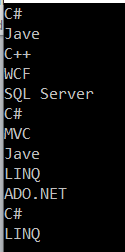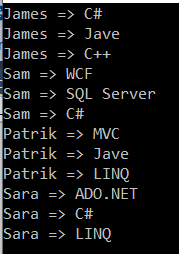# SelectMany in LINQ

## SelectMany in LINQ with Examples

In this article, I am going to discuss the SelectMany in LINQ with examples. Please read our previous article where we discussed the Select Operator in C# with some examples. The SelectMany Method belongs to the projection category operator. As part of this article, we are going to discuss the following pointers in details.

1. What is SelectMany Operator in LINQ?
2. Examples using both query and method syntax in C#.
##### What is SelectMany Operator in LINQ?

The SelectMany in LINQ is used to project each element of a sequence to an IEnumerable<T> and then flatten the resulting sequences into one sequence. That means the SelectMany operator combines the records from a sequence of results and then converts it into one result.

If this is not clear at the moment, then don’t worry we will see it in practice.

##### Example1:

Let us first write a program and see the output then we will discuss how it will work.

```using System;
using System.Collections.Generic;
using System.Linq;

namespace LINQDemo
{
class Program
{
static void Main(string[] args)
{
List<string> nameList =new List<string>(){"Pranaya", "Kumar" };
IEnumerable<char> methodSyntax = nameList.SelectMany(x => x);
foreach(char c in methodSyntax)
{
Console.Write(c + " ");
}

}
}
}```
##### Let us understand the above code:

In the above code, we see that the SelectMany method returns an IEnumerable<char>. This is because the SelectMany method returns all the elements from the sequence. Here the List is the sequence. And the list contains the strings. Here the list contains two strings. So, the SelectMany method fetches all the characters from the above two strings and then converts it into one sequence i.e. IEnumerable<char>.

So, when we execute the above program, it will give us the following output.##### SelectMany Operator Using Query Syntax in C#.NET:

The most important point is that there is no such SelectMany operator available in LINQ to write query syntax. But we can achieve this by writing multiple “from clause” in the query as shown in the below example.

```using System;
using System.Collections.Generic;
using System.Linq;

namespace LINQDemo
{
class Program
{
static void Main(string[] args)
{
List<string> nameList =new List<string>(){"Pranaya", "Kumar" };

IEnumerable<char> querySyntax = from str in nameList
from ch in str
select ch;

foreach (char c in querySyntax)
{
Console.Write(c + " ");
}

}
}
}
```

Now, execute the program and you will see the same output as method syntax as expected.

##### Example2: LINQ SelectMany Operator with Complex Type

Let us create a class file with the name Student.cs and then copy and paste the following code.

```using System.Collections.Generic;
namespace LINQDemo
{
public class Student
{
public int ID { get; set; }
public string Name { get; set; }
public string Email { get; set; }
public List<string> Programming { get; set; }

public static List<Student> GetStudents()
{
return new List<Student>()
{
new Student(){ID = 1, Name = "James", Email = "James@j.com", Programming = new List<string>() { "C#", "Jave", "C++"} },
new Student(){ID = 2, Name = "Sam", Email = "Sara@j.com", Programming = new List<string>() { "WCF", "SQL Server", "C#" }},
new Student(){ID = 3, Name = "Patrik", Email = "Patrik@j.com", Programming = new List<string>() { "MVC", "Jave", "LINQ"} },
new Student(){ID = 4, Name = "Sara", Email = "Sara@j.com", Programming = new List<string>() { "ADO.NET", "C#", "LINQ" } }
};
}
}
}
```

As you can see we have created the Student class with four properties. Please remember the Programming property returns list<string>. Here we also created one method which will return the List of students which will be going to act our data source.

We need to Projects all programming strings of all the students to a single IEnumerable<string>. As you can see, we have 4 students, so there will be 4 IEnumerable<string> sequences, which then we need to flattened to form a single sequence i.e. a single IEnumerable<string> sequence.The code is given below.

```using System;
using System.Collections.Generic;
using System.Linq;

namespace LINQDemo
{
class Program
{
static void Main(string[] args)
{
//Using Method Syntax
List<string> MethodSyntax = Student.GetStudents().SelectMany(std => std.Programming).ToList();

//Using Query Syntax
IEnumerable<string> QuerySyntax = from std in Student.GetStudents()
from program in std.Programming
select program;

//Printing the values
foreach (string program in MethodSyntax)
{
Console.WriteLine(program);
}

}
}
}
```

When we execute the program, it gives us the following output.##### Example3:

In our previous example, we get the output as expected but with the duplicate program names. If you want only the distinct program names then you need to apply the distinct method on the result set as shown in the below examples.

```using System;
using System.Collections.Generic;
using System.Linq;

namespace LINQDemo
{
class Program
{
static void Main(string[] args)
{
//Using Method Syntax
List<string> MethodSyntax = Student.GetStudents()
.SelectMany(std => std.Programming)
.Distinct()
.ToList();

//Using Query Syntax
IEnumerable<string> QuerySyntax = (from std in Student.GetStudents()
from program in std.Programming
select program).Distinct().ToList();

//Printing the values
foreach (string program in QuerySyntax)
{
Console.WriteLine(program);
}

}
}
}```

Now execute the program and you will see that it will remove the duplicate program names from the sequence.

##### Example4:

Now we need to retrieve the student name along with the program language name.

```using System;
using System.Collections.Generic;
using System.Linq;

namespace LINQDemo
{
class Program
{
static void Main(string[] args)
{
//Using Method Syntax
var MethodSyntax = Student.GetStudents()
.SelectMany(std => std.Programming,
(student, program) => new
{
StudentName = student.Name,
ProgramName = program
}
)
.ToList();

//Using Query Syntax
var QuerySyntax = (from std in Student.GetStudents()
from program in std.Programming
select new {
StudentName = std.Name,
ProgramName = program
}).ToList();

//Printing the values
foreach (var item in QuerySyntax)
{
Console.WriteLine(item.StudentName + " => " + item.ProgramName);
}